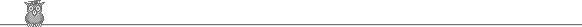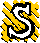### 52nd Putnam 1991A1.  The rectangle with vertices (0, 0), (0, 3), (2, 0) and (2, 3) is rotated clockwise through a right angle about the point (2, 0), then about (5, 0), then about (7, 0), and finally about (10, 0). The net effect is to translate it a distance 10 along the x-axis. The point initially at (1, 1) traces out a curve. Find the area under this curve (in other words, the area of the region bounded by the curve, the x-axis and the lines parallel to the y-axis through (1, 0) and (11, 0) ).A2.  M and N are real unequal n x n matrices satisfying M3 = N3 and M2N = N2M. Can we choose M and N so that M2 + N2 is invertible?A3.  P(x) of is a polynomial of degree n ≥ 2 with real coefficients, such that (1) it has n unequal real roots, (2) for each pair of adjacent roots a, b the derivative P'(x) is zero halfway between the roots (at x = (a + b)/2 ). Find all possible P(x).A4.  Can we find an (infinite) sequence of disks in the Euclidean plane such that: (1) their centers have no (finite) limit point in the plane; (2) the total area of the disks is finite; and (3) every line in the plane intersects at least one of the disks?A5.  Let f(z) = ∫0z   √(x4 + (z - z2)2) dx. Find the maximum value of f(z) in the range 0 ≤ z ≤ 1.A6.  An n-sum of type 1 is a finite sequence of positive integers a1, a2, ... , ar, such that: (1) a1 + a2 + ... ar = n; and (2)   a1 > a2 + a3,   a2 > a3 + a4, ... , ar-2 > ar-1 + ar, and ar-1 > ar. For example, there are five 7-sums of type 1, namely: 7; 6, 1; 5, 2; 4, 3; 4, 2, 1. An n-sum of type 2 is a finite sequence of positive integers b1, b2, ... , bs such that: (1) b1 + b2 + ... + bs = n; (2) b1 ≥ b2 ≥ ... ≥ bs; (3) each bi is in the sequence 1, 2, 4, ... , gj, ... defined by g1 = 1, g2 = 2, gj = gj-1 + gj-2 + 1; and (4) if b1 = gk, then 1, 2, 4, ... , gk is a subsequence. For example, there are five 7-sums of type 2, namely: 4, 2, 1; 2, 2, 2, 1; 2, 2, 1, 1, 1; 2, 1, 1, 1, 1, 1; 1, 1, 1, 1, 1, 1, 1. Prove that for n ≥ 1 the number of type 1 and type 2 n-sums is the same.B1.  For positive integers n define d(n) = n - m2, where m is the greatest integer with m2 ≤ n. Given a positive integer b0, define a sequence bi by taking bk+1 = bk + d(bk). For what b0 do we have bi constant for sufficiently large i?B2.  R is the real line. f, g: R -> R are non-constant, differentiable functions satisfying: (1) f(x + y) = f(x)f(y) - g(x)g(y) for all x, y; (2) g(x + y) = f(x)g(y) + g(x)f(y) for all x, y; and (3) f '(0) = 0. Prove that f(x)2 + g(x)2 = 1 for all x.B3.  Can we find N such that all m x n rectangles with m, n > N can be tiled with 4 x 6 and 5 x 7 rectangles?B4.  p is a prime > 2. Prove that ∑0≤n≤p pCn (p+n)Cn = 2p + 1 (mod p2). [aCb is the binomial coefficient a!/(b! (a-b)!).]B5.  p a prime > 2. How many residues mod p are both squares and squares plus one?B6.  Let a and b be positive numbers. Find the largest number c, in terms of a and b, such that for all x with 0 < |x| ≤ c and for all α with 0 < α < 1, we have:   aα b1-α ≤ a sinh αx/sinh x + b sinh x(1 - α)/sinh x.To avoid possible copyright problems, I have changed the wording, but not the substance of all the problems. The original text of the problems and the official solutions are in American Mathematical Monthly 99 (1991) 717-724.

Putnam home